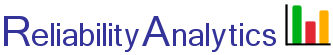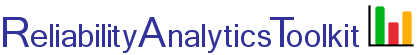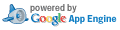# Weibull Distribution

The Weibull distribution can be used to model many different failure distributions. Given a shape parameter (β) and characteristic life (η) the reliability can be determined at a specific point in time (t). The two-parameter Weibull distribution probability density function, reliability function and hazard rate are given by: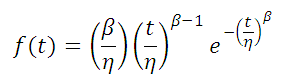Probability Density Function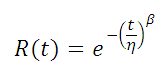Reliability Function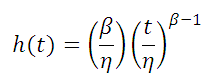Hazard Rate

The characteristic life (η) is the point where 63.2% percent of the population will have failed, regardless of the shape parameter (β). The following shape parameter characteristics are noted:

β = 1.0 : Exponential distribution, constant failure rate
β = 3.5 : Normal distribution (approximation)
β < 1.0 : Decreasing failure (hazard) rate
β > 1.0 : Increasing failure (hazard) rate

## Calculation Inputs:

1. Shape parameter (β):
2. Characteristic life (η):
3. Time period of interest (t):
4. Units associated with inputs #2 and #3 above:
5. Decimal places:
6. Options:

Calculate mean life
Table
f(t) chart
R(t) chart
F(t) chart
h(t) chart

Title:

## Featured Reference: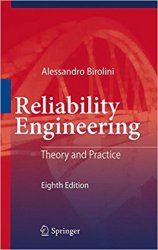Reliability Engineering: Theory and Practice

Toolkit Home